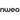# Negative Exponents: 8 Things Your Students Need To Know

Maria Kampen

All Posts
Many students already struggle to understand negative numbers, exponent rules and fractions. So what happens when you add negative exponents to the equation?Total chaos. Well, not really. But understanding negative exponents is an important building block for high school-level math courses, and it’s also a concept many students find challenging. When you gradually build on your students’ knowledge, you’ll ensure they’re ready to tackle challenging problems in and out of the classroom.If you’re not sure where to begin, this blog post will help you transform your unit on negative exponents into a positive experience for you and your students!We’ll cover:

## Negative exponents rulesLike everything else in math class, negative exponents have to follow rules. If you need a reminder , here’s a quick recap of the seven rules of exponents:
1. Product of powers: Add powers together when multiplying like bases
2. Quotient of powers rule: Subtract powers when dividing like bases
3. Power of powers rule: Multiply powers together when raising a power by another exponent
4. Power of a product rule: Distribute power to each base when raising several variables by a power
5. Power of a quotient rule: Distribute power to each base when raising several variables by a power
6. Zero power rule: Any base raised to the power of zero becomes one
7. Negative exponent rule: To change a negative exponent to a positive one, flip it into a reciprocal.
Remind students that the rules stay the same with negative exponents — there just might be a few extra steps to follow.

## A quick review of negative numbers

Negative numbers require a certain amount of abstract thinking that doesn’t always come naturally. But without a solid understanding of negative numbers, students won’t be ready to tackle negative exponents.Here’s a quick review:A negative number is any number less than zero. Negative numbers are expressed with a negative sign. For example, -4 is four less than zero. It’s helpful to think of negative numbers as existing on a number line: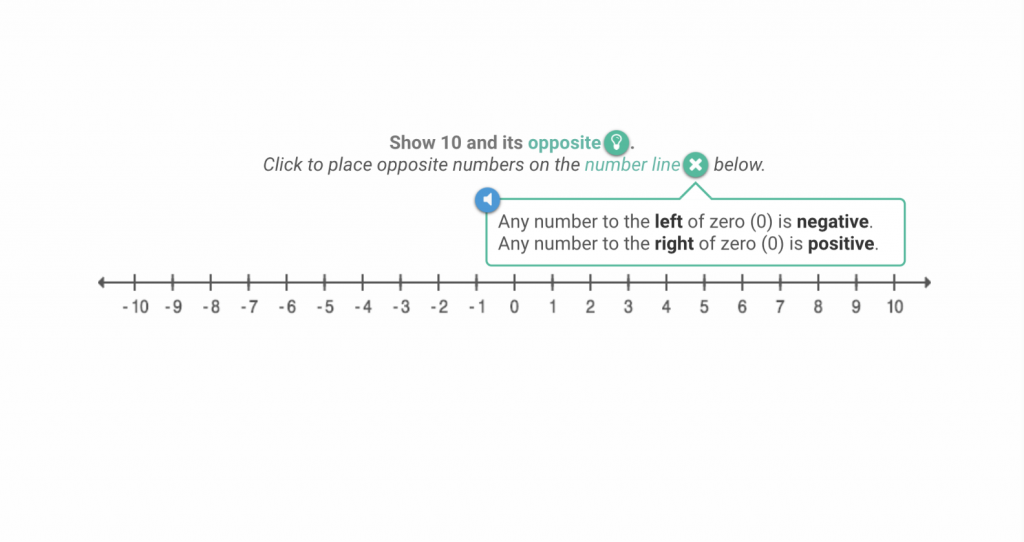When you add and subtract negative numbers, you’re either moving to the right or the left of the number line. When you subtract a negative number you move to the left of the number line, because it’s the same as adding a positive number. If you’re adding a negative number you move to the right because it’s the same as subtracting a positive number.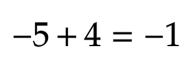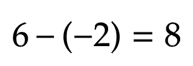When you multiply a negative number by a positive number (or vice versa), the product will be negative. If you multiply two negative numbers or two positive numbers, the result will be positive.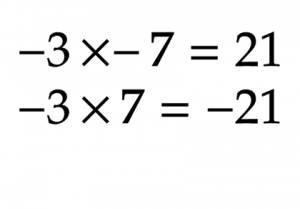Multiplying different signs will always give you a negative product, and multiplying the same signs will give you a positive product. Always assume the number is positive if there’s no sign in front of it.

## What do negative exponents mean?

We already know that positive exponents are a way of expressing repeated multiplication. For example: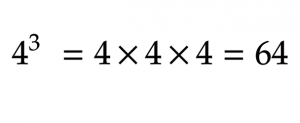There are a few different ways of thinking about negative exponents, but in general, negative exponents are the opposite of positive ones.

|

All negative exponents can be expressed as their positive reciprocal. A reciprocal is a fraction where the numerator and denominator switch places.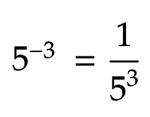How can something be flipped into a reciprocal if it wasn’t a fraction to start?We know that numbers can be expressed in more than one way. For example, eight can also be written as: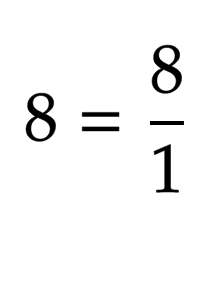So, negative exponents can be expressed as the positive reciprocal of the base multiplied by itself x times.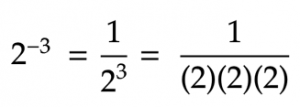The larger the negative exponent, the smaller the number it represents. While positive exponents indicate repeated multiplication, negative exponents represent repeated division. That’s why 2-3 is greater than 2-6.

|

## How to solve negative exponents

Most questions will ask you to solve negative exponents by expressing them as positive equations. Here’s how: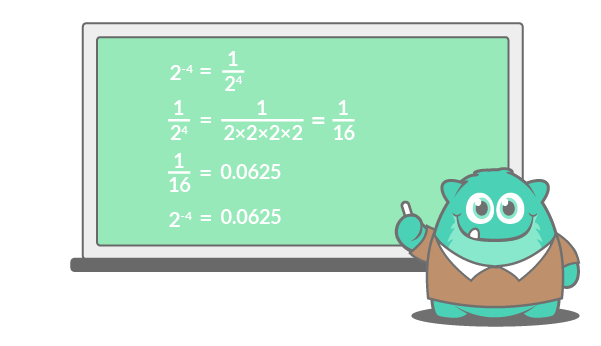Flip the base and exponent into the reciprocal, then solve the denominator. Divide the numerator by the denominator to find the final decimal.

## Multiplying and dividing negative exponents

We’ve already covered multiplying exponents, but here’s a quick review on how to multiply and divide negative exponents.

### Multiplying negative exponents

Good news! The rules for multiplying exponents are the same, even when the exponent is negative. If the bases are the same, add the exponents. Remember to keep in mind the rules for adding and subtracting negative numbers.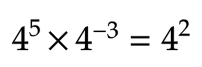If the bases are different but the exponents are the same, multiply the bases and leave the exponents the way they are.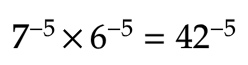If there’s nothing in common, go directly to solving the equation. Flip the exponents into their reciprocals, then multiply.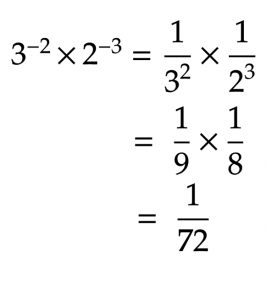If you need a reminder, check out our post on How to Multiply Fractions.

### Dividing negative exponents

Dividing negative exponents is almost the same as multiplying them, except you’re doing the opposite: subtracting where you would have added and dividing where you would have multiplied. If the bases are the same, subtract the exponents.Remember to flip the exponent and make it positive, if needed.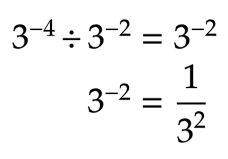If the exponents are the same but the bases are different, divide the bases first.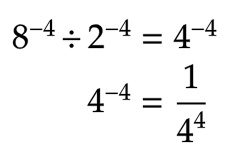If there’s nothing in common, go directly to solving the equation.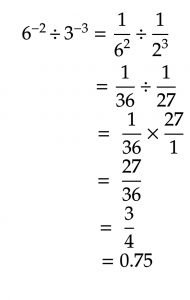For a refresher on dividing fractions, check out our How to Divide Fractions blog post.

## Negative numbers with exponentsWhat happens if the base is negative instead of the exponents?If the exponent is positive, work with it as you would a regular exponent, but remember two things:
• If the base is negative and the exponent is an even number, the final product will always be a positive number.
• If the base is negative and the exponent is an odd number, the final product will always be a negative number.
If there are parentheses around the negative base, the power applies to the entire equation -- including the negative sign. If there are no parentheses, the power applies only to the base and not to the negative sign.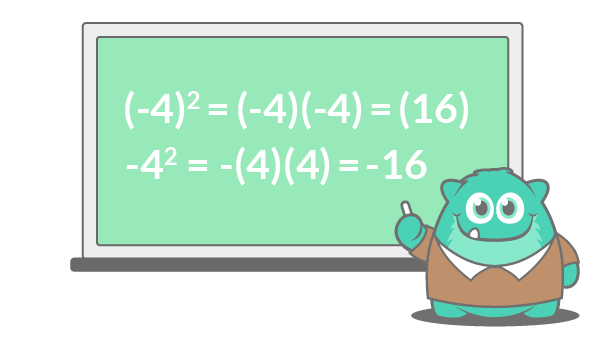Since the first example is being raised to an even power, the two negative signs cancel out and you’re left with a positive product. If the exponent was an odd power, the product would be negative because there would be one number that couldn’t cancel out.In the second example, the positive power only applies to the four, not to the negative sign. In this case, the negative sign tells you the product will be negative whether the power is odd or even.

## Simplifying negative exponents

Multiplying, dividing and understanding negative exponents is the first step to simplifying expressions with negative exponents. Remember: all the steps covered above hold true no matter how complicated the expression is. Let’s start by multiplying negative exponents with variables.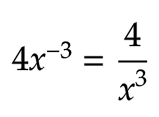In this example, the power only applies to the x base, not the 4. To make it a positive expression, flip the x to the reciprocal and keep the 4 on top. Let’s try something a little more complicated.The variables here are the same, so according to the first exponent rule, we can multiply the numbers, keep the base the same and add the exponents together.Multiply the 6 and 4 for a product of 24. Then, add the exponents together to multiply the x variables.What about dividing negative exponents with variables? Let’s start with a simple example: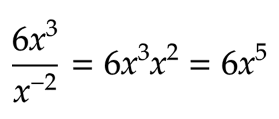To make the negative exponent positive, move the ???? to the top of the equation and multiply. Here’s an example of negative exponents with multiple variables: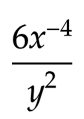Since the negative exponent only applies to the variable, move 𝑥-4 to the bottom of the equation to make it positive, and leave the 6 where it is.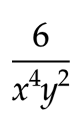And there’s your simplified equation! Let’s try another one.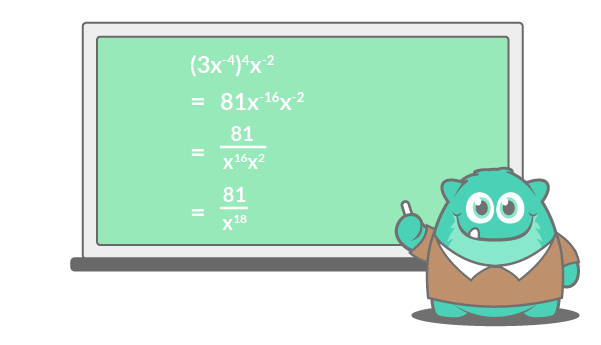First, redistribute the power to the inside of the brackets, following the third exponent rule.Then, flip the 𝑥 variables with negative exponents to their reciprocal.Finally, multiply the 𝑥 variables by adding the exponents together.Let’s do one more.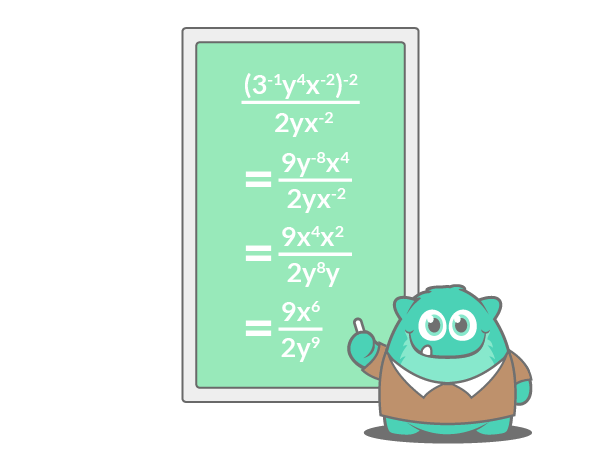To start, either square the equation or move the parentheses first. We’ll begin by squaring the top bracket and redistributing the power. Then, move the negative exponents down or up, depending on their positions. A negative exponent on top can be brought to the bottom so it’s a reciprocal, and vice versa. Finish by simplifying.There’s often more than one way to simplify negative exponent expressions. Since exponents are repeated multiplication, and you can multiply numbers in whatever order you’d like, different steps can lead to the same result.

## Fractions with negative exponents

We know what to do with whole numbers that have negative exponents, but what about fractions with negative exponents?To simplify fractions with negative exponents, flip them to their reciprocals, multiply and reduce.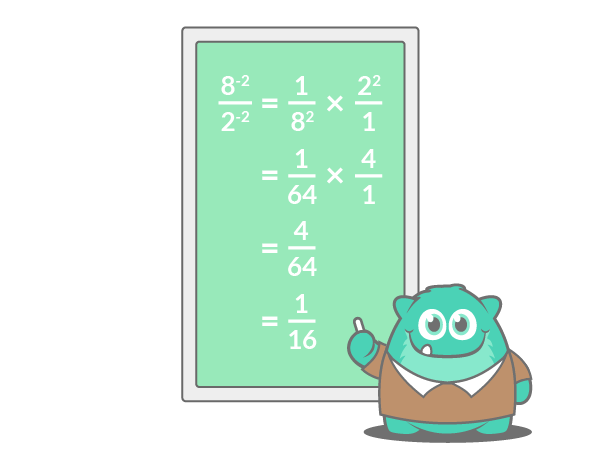## How to teach negative exponents with Prodigy

Students will love practicing negative exponents with Prodigy: a free, curriculum-aligned math platform packed with exotic pets, fun challenges and educational adventure. Negative exponents are an important concept for students to grasp before they start high school, but many learners struggle with key concepts. Using powerful reporting tools in your Teacher Dashboard, you’ll see which topics your students have mastered, and where they need more practice.Prodigy’s Assignments, Plans and Test Prep features let you assign targeted math practice for students who are struggling or excelling. You’ll get real-time data as students play, and you’ll be able to deliver differentiated assignments that align with what you’re teaching in class.You can use Prodigy to:Best of all? These tools are completely free for teachers and students. To learn more about aligning Prodigy to your classroom, find out how you can use customized Plans to strengthen your lesson content.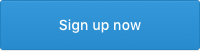## Final thoughts on negative exponents

If you’d like more practice on exponents in general, our exponent rules worksheet gives students the opportunity to get more familiar with how exponents work.When working with negative exponents, it’s important to remember all the exponent rules stay the same. Besides this, the only thing students need to know is how to add, subtract, multiply and divide negative numbers. Take it slow, and build up to more complicated questions. Your students will be exponent whizzes in no time at all!
Start teaching negative exponents with Prodigy today. Prodigy is a no-cost, curriculum-aligned math platform that encourages students to love learning math. With over a million teachers and 50 million students, Prodigy offers unique solutions for your classroom.•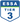•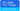•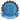•